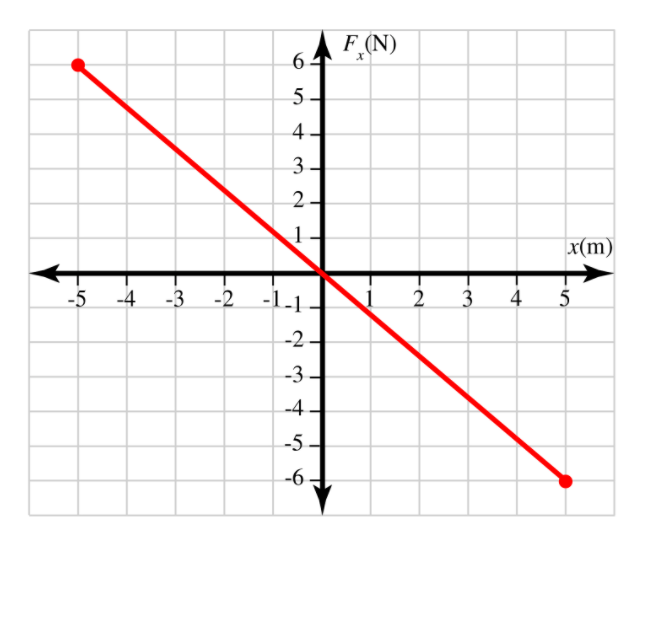# Question A block of mass 𝑚=4.43 kgm=4.43 kg is attached to a spring that is resting on a horizontal, frictionless table. The block is pushed into the spring, compressing it by 5.00 m5.00 m, and is then released from rest. The spring begins to push the block back toward the equilibrium position at 𝑥=0 mx=0 m. The graph shows the component of the force (in newtons) exerted by the spring on the block versus the position of the block (in meters) relative to equilibrium. Use the graph to answer the questions. How much work 𝑊W is done by the spring in pushing the block from its initial position at 𝑥=−5.00 mx=−5.00 m to 𝑥=2.42 mx=2.42 m? F (N) 6 5 4 3 2 1 x(m) 5 -5 -4 -3 -2 -1.1 2 3 4 5 -2 - -3 -4 -5 -6A block of mass 𝑚=4.43 kgm=4.43 kg is attached to a spring that is resting on a horizontal, frictionless table. The block is pushed into the spring, compressing it by 5.00 m5.00 m, and is then released from rest. The spring begins to push the block back toward the equilibrium position at 𝑥=0 mx=0 m. The graph shows the component of the force (in newtons) exerted by the spring on the block versus the position of the block (in meters) relative to equilibrium. Use the graph to answer the questions.